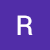# Introduction:

There are a number of algorithms which help us to reduce time complexity or space complexity of programs and divide and conquer is one of them. Divide and conquer algorithm has been here for ages and can be traced back to as far as the 200 BC in Babylonia. Historical examples of the use of the algorithm include Cooley–Tukey fast Fourier transform (FFT), binary search, merge sort and the Euclidean algorithm to compute the greatest common divisor of two numbers. As the name suggests, divide and conquer algorithm can be understood in three simple steps, that is:

1. Divide

2…

# Demystifying LSTM

INTRODUCTION:

Let’s start with the basics. Long Short Term Memory models (henceforth, referred as LSTM) are special types of recurrent neural networks (or RNN, for short). RNNs are algorithms that are best suited for sequential data, as they can remember the input given due to their internal memory. LSTMs are a better (read, modified) version of RNNs which are suited for time series forecasting. Some major terminologies (and their definitions) you should be aware of before proceeding with the article:

· Neural networks: A set of algorithms designed to resemble the human brain as much as possible and to recognize…